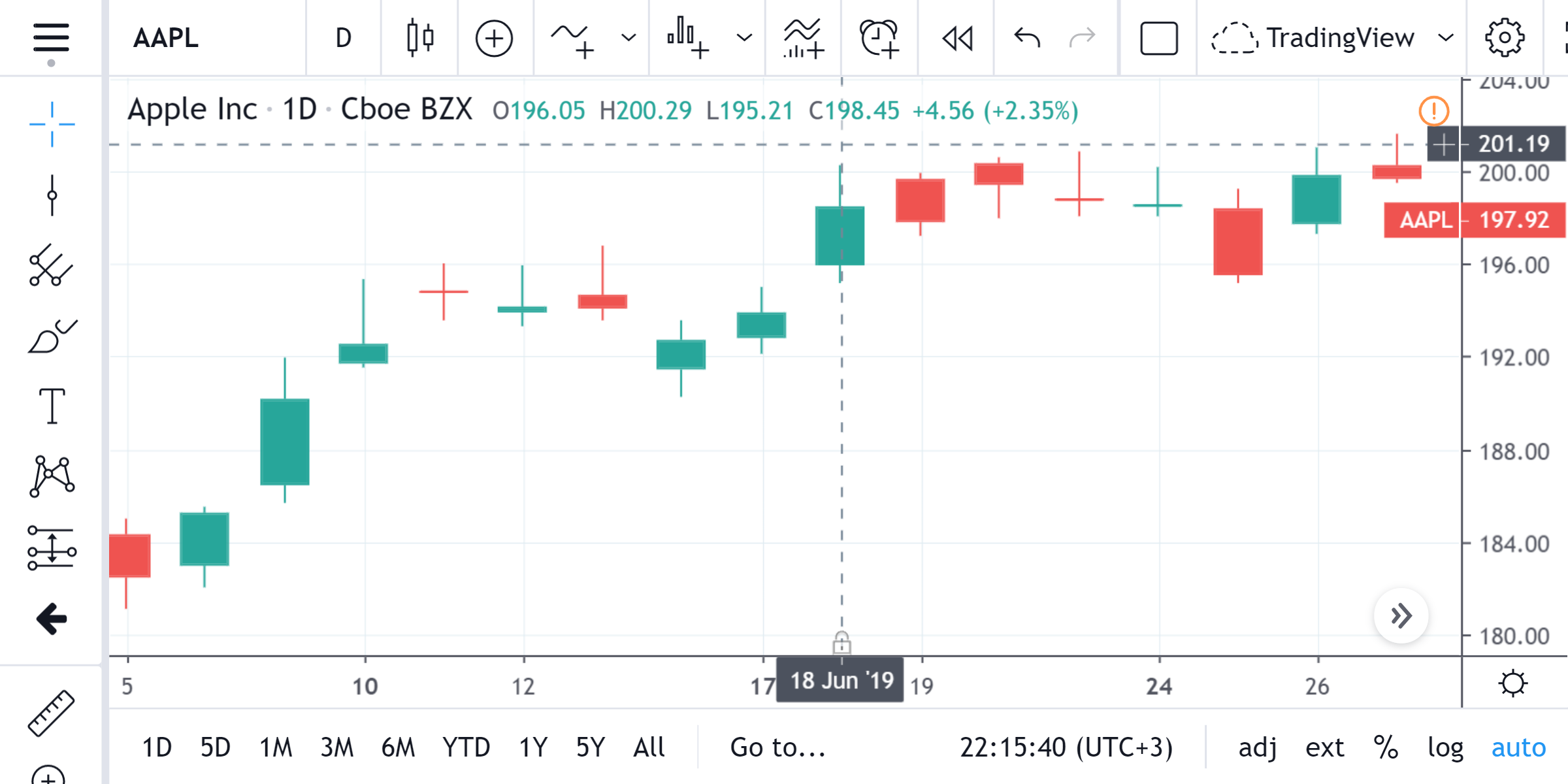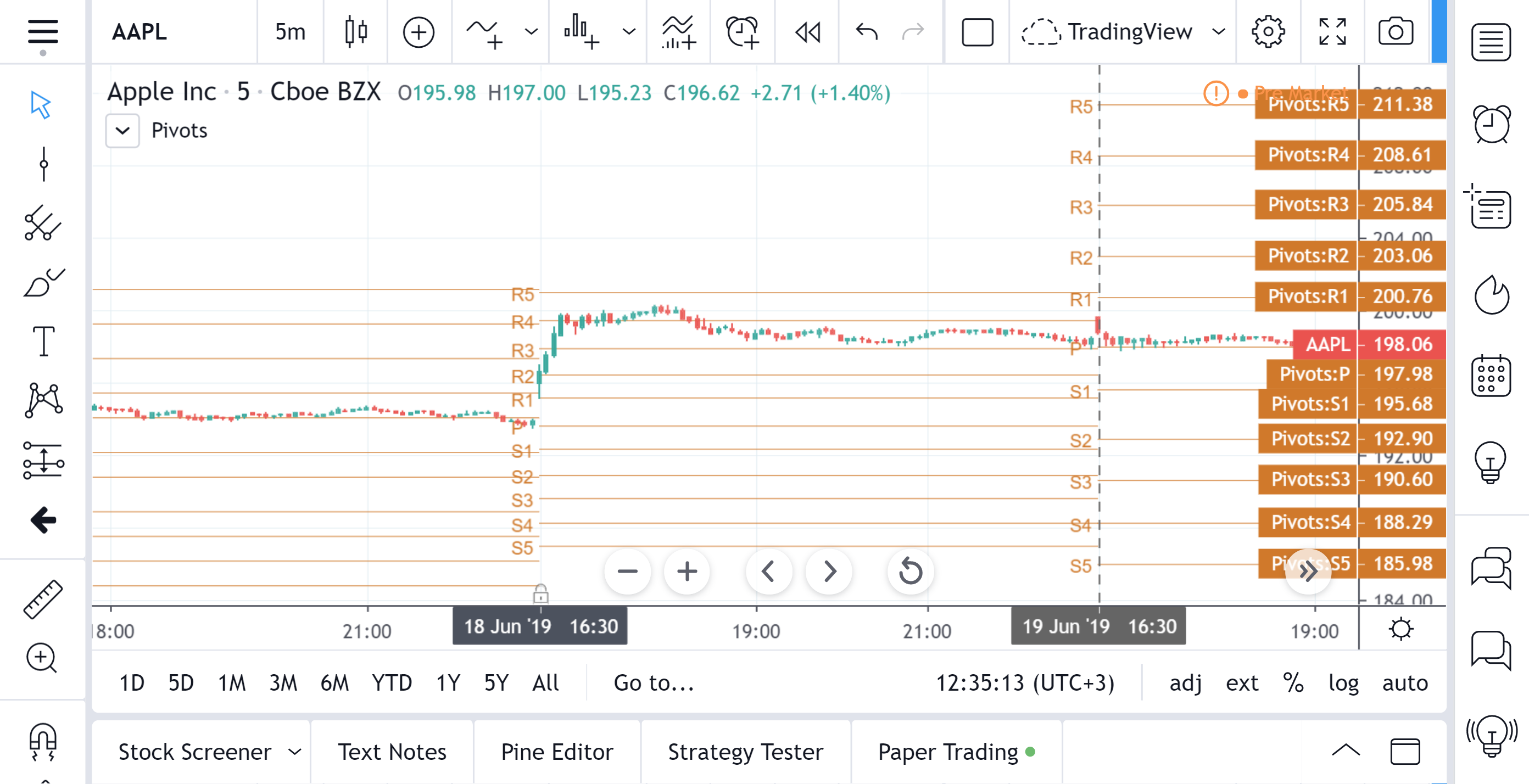# Pivot Points Standard

Pivot Points Standard — is a technical indicator that is used to determine the levels at which price may face support or resistance. The Pivot Points indicator consists of a pivot point (PP) level and several support (S) and resistance (R) levels.

#### Calculation

PP, resistance and support values are calculated in different ways, depending on the type of the indicator, specified by the Type field in indicator inputs. To calculate PP and support/resistance levels, the values OPENcurr, OPENprev, HIGHprev, LOWprev, CLOSEprev are used, which are the values of the current open and previous open, high, low and close, respectively, on the indicator resolution. The indicator resolution is set by the input of the Pivots Timeframe. If the Pivots Timeframe is set to AUTO (the default value), then the increased resolution is determined by the following algorithm:

• for intraday resolutions up to and including 15 min, DAY (1D) is used
• for intraday resolutions more than 15 min, WEEK (1W) is used
• for daily resolutions MONTH is used (1M)
• for weekly and monthly resolutions, 12-MONTH (12M) is used

#### Types

Tradingview uses the following types of the Pivot Points indicator:

• Fibonacci
• Woodie
• Classic
• Demark
• Camarilla

The calculation formulas for each type are given below.

``````    PP = (HIGHprev + LOWprev + CLOSEprev) / 3
R1 = PP * 2 - LOWprev
S1 = PP * 2 - HIGHprev
R2 = PP + (HIGHprev - LOWprev)
S2 = PP - (HIGHprev - LOWprev)
R3 = PP * 2 + (HIGHprev - 2 * LOWprev)
S3 = PP * 2 - (2 * HIGHprev - LOWprev)
R4 = PP * 3 + (HIGHprev - 3 * LOWprev)
S4 = PP * 3 - (3 * HIGHprev - LOWprev)
R5 = PP * 4 + (HIGHprev - 4 * LOWprev)
S5 = PP * 4 - (4 * HIGHprev - LOWprev)``````
###### Fibonacci
``````    PP = (HIGHprev + LOWprev + CLOSEprev) / 3
R1 = PP + 0.382 * (HIGHprev - LOWprev)
S1 = PP - 0.382 * (HIGHprev - LOWprev)
R2 = PP + 0.618 * (HIGHprev - LOWprev)
S2 = PP - 0.618 * (HIGHprev - LOWprev)
R3 = PP + (HIGHprev - LOWprev)
S3 = PP - (HIGHprev - LOWprev)``````
###### Woodie
``````    PP = (HIGHprev + LOWprev + 2 * OPENcurr) / 4
R1 = 2 * PP - LOWprev
S1 = 2 * PP - HIGHprev
R2 = PP + (HIGHprev - LOWprev)
S2 = PP - (HIGHprev - LOWprev)
R3 =  HIGHprev + 2 * (PP -  LOWprev)
S3 =  LOWprev - 2 * (HIGHprev - PP)
R4 = R3 + (HIGHprev - LOWprev)
S4 = S3 - (HIGHprev - LOWprev)``````
###### Classic
``````    PP = (HIGHprev + LOWprev + CLOSEprev) / 3
R1 = 2 * PP - LOWprev
S1 = 2 * PP - HIGHprev
R2 = PP + (HIGHprev - LOWprev)
S2 = PP - (HIGHprev - LOWprev)
R3 = PP + 2 * (HIGHprev - LOWprev)
S3 = PP - 2 * (HIGHprev - LOWprev)
R4 = PP + 3 * (HIGHprev - LOWprev)
S4 = PP - 3 * (HIGHprev - LOWprev)``````
###### Demark
``````    IF  OPENprev == CLOSEprev
X = HIGHprev + LOWprev + 2 * CLOSEprev
ELSE
IF CLOSEprev >  OPENprev
X = 2 * HIGHprev + LOWprev + CLOSEprev
ELSE
X = 2 * LOWprev + HIGHprev + CLOSEprev
PP = X / 4
R1 = X / 2 - LOWprev
S1 = X / 2 - HIGHprev``````
###### Camarilla
``````    PP = (HIGHprev + LOWprev + CLOSEprev) / 3
R1 = CLOSEprev + 1.1 * (HIGHprev - LOWprev) / 12
S1 = CLOSEprev - 1.1 * (HIGHprev - LOWprev) / 12
R2 = CLOSEprev + 1.1 * (HIGHprev - LOWprev) / 6
S2 = CLOSEprev - 1.1 * (HIGHprev - LOWprev) / 6
R3 = CLOSEprev + 1.1 * (HIGHprev - LOWprev) / 4
S3 = CLOSEprev - 1.1 * (HIGHprev - LOWprev) / 4
R4 = CLOSEprev + 1.1 * (HIGHprev - LOWprev) / 2
S4 = CLOSEprev - 1.1 * (HIGHprev - LOWprev) / 2
R5 = (HIGHprev / LOWprev) * CLOSEprev
S5 = CLOSEprev - (R5 - CLOSEprev)``````

Calculation example

Input: NASDAQ:AAPL, 5 minutes timeframe. We calculate the Pivot Points values on June 19, 2019 by choosing the following inputs:

• Pivots Timeframe: Auto

First, let's determine the indicator resolution (the algorithm is described above). Resolution of the chart is 5 minutes, i.e. intraday less than 15 minutes, so the indicator resolution is 1D. Therefore, we obtain the values of the high, low, close series for the previous day, i.e. June 18, 2019:HIGHprev = 200.29
LOWprev = 195.21
CLOSEprev = 198.45

We calculate the values of PP, S, and P according to the formula for the Traditional type:

``````    PP = (HIGHprev + LOWprev + CLOSEprev) / 3
R1 = PP * 2 - LOWprev
S1 = PP * 2 - HIGHprev
R2 = PP + (HIGHprev - LOWprev)
S2 = PP - (HIGHprev - LOWprev)
R3 = PP * 2 + (HIGHprev - 2 * LOWprev)
S3 = PP * 2 - (2 * HIGHprev - LOWprev)
R4 = PP * 3 + (HIGHprev - 3 * LOWprev)
S4 = PP * 3 - (3 * HIGHprev - LOWprev)
R5 = PP * 4 + (HIGHprev - 4 * LOWprev)
S5 = PP * 4 - (4 * HIGHprev - LOWprev)``````
``````    PP = (200.29 + 195.21 + 198.45) / 3 = 197.983333333
R1 = 197.983333333 * 2 - 195.21 = 200.756666666
S1 = 197.983333333 * 2 - 200.29 = 195.676666666
R2 = 197.983333333 + (200.29 - 195.21) = 203.063333333
S2 = 197.983333333 - (200.29 - 195.21) = 192.903333333
R3 = 197.983333333 * 2 + (200.29 - 2 * 195.21) = 205.836666666
S3 = 197.983333333 * 2 - (2 * 200.29 - 195.21) = 190.596666666
R4 = 197.983333333 * 3 + (200.29 - 3 * 195.21) = 208.609999999
S4 = 197.983333333 * 3 - (3 * 200.29 - 195.21) = 188.289999999
R5 = 197.983333333 * 4 + (200.29 - 4 * 195.21) = 211.383333332
S5 = 197.983333333 * 4 - (4 * 200.29 - 195.21) = 185.983333332``````Minor differences in values may be due to specifics of rounding.